## Statistics Sampler

Jim Mahoney, September 2003

### •Looking around

To enter a table from the keyboard, use control-comma to add another column and control-return to add another row.  The grouping symbols at the left and right sides are just parenthesis which grow as needed.  Use tab to move to the next entry and control-space to move the cursor out of the table.  Mathematica stores this data as a list of lists.  Non-numeric data must be enclosed by double quotes.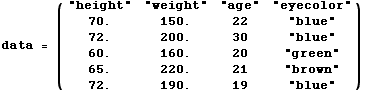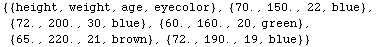We can save this table to a .csv (comma separated values).  Like most computer applications, Mathematica has the notion of a "current directory" where files are put.  Use SetDirectory["name"] to move around.
To read this data in we'd type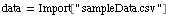.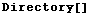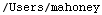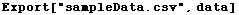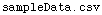Here's how we'd extract some values into a list.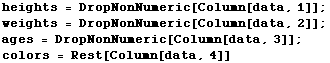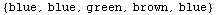There are also many ways to make pictures from the data.  Here are a couple simple examples.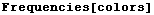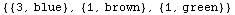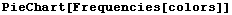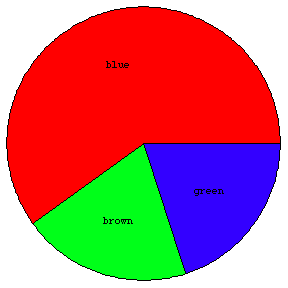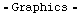This next one shows how options are added.  The arrow is typed as ->.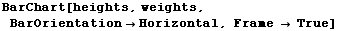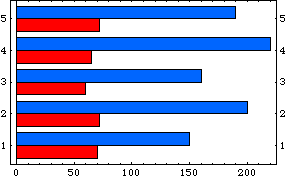And we can evaluate various statistical formulas.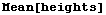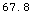Mathematica has verbose and not always obvious names for the formulas in the text.  The one for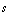, which estimates a population standard deviation from a given sample is called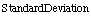.  The actual formula that this calculates is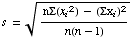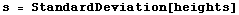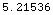If on the other had we want the pure standard deviation of this list, treating it as the entire population, then the formula is slightly different.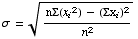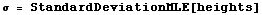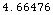Note that to type the symbol σ (sigma) from the keyboard,type use the escape key to type esc-s-esc or esc-sigma-esc.Likewise the symbol Σ (capital Sigma) is typed as esc-S-esc or esc-Sigma-esc.

Here's a summary of a variety of the descriptive statistics.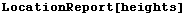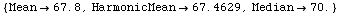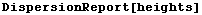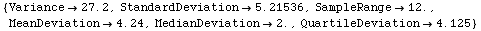One quirk of Mathematica is that it doesn't convert to decimal numbers unless necessary.  The ages in the table above were given as integer values without a decimal point, and so the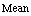formula gives this result.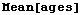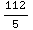To see a decimal value for an expression like this, append //N (which means Number) to the end.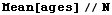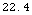Converted by Mathematica  (September 11, 2003)